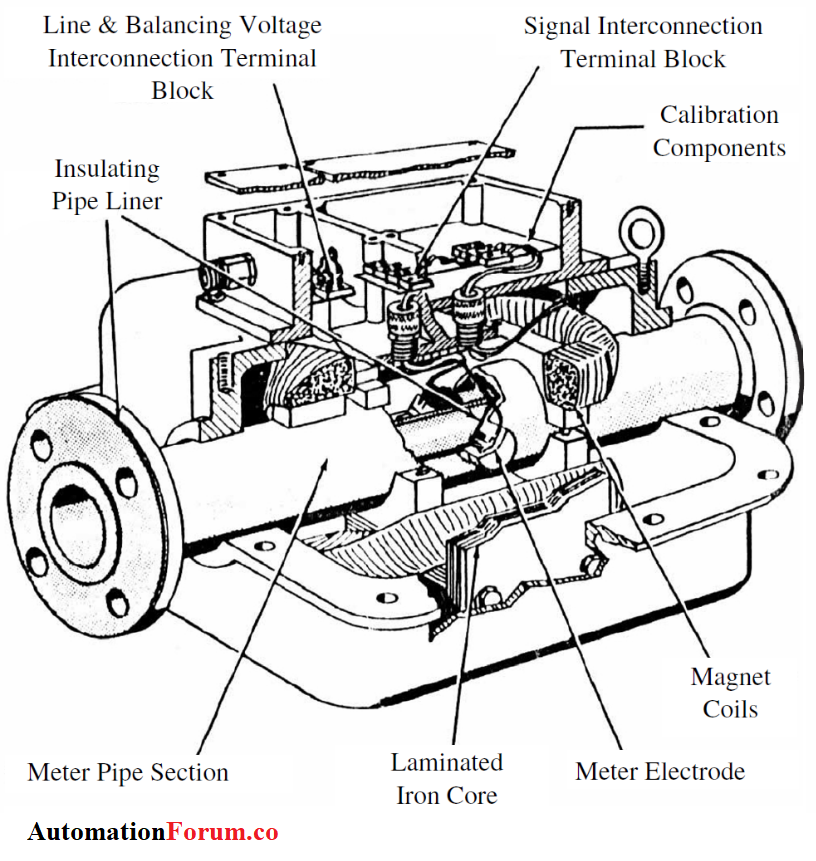# Construction of Electromagnetic flowmeter### Working principle of electromagnetic flowmeter:

In the electromagnetic meter, the constant magnetic field is generated around the pipe by the magnet and the flowing liquid acts as a conductor.

The fluid that flows can be considered as a continuous series of disks that pass through the magnetic field, with the orifice of the tube directly proportional to the length of the conductor. Therefore, the generated emf is directly proportional to the flow velocity.

## Construction of Electromagnetic flowmeter:

The basic element flowmeter are – a non conducting pipe or a nonmagnetic pipe section, On alternate sides of the pipe section are magnet coils which produce the magnetic field perpendicular to the flow of liquid through the pipe and in contact with the liquid, is a pair of electrodes which are located at right angles both to the magnetic field and the axis of the pipe.

As the liquid passes through the section of the pipe, it also passes through the magnetic field’s configuration through the coils of the magnet, which induces a voltage in the liquid; The amplitude of the voltage is directly proportional to the speed of the liquid.

This voltage is conducted by the electrodes to a separate converter which in effect is a precision voltmeter capable of precisely measuring the voltage generated and converting that voltage into the desired control signals.

## AC and DC Excitation:

Magnetic flow meters type AC line voltage is applied to the magnetic coils. The signal generated is a low level AC signal in the range of high μV to low mV.

And there is DC pulsed DC type magnetic flow meter. In such flow meters, the coils are periodically energized.

There are many forms of excitation in use, but generally they can be categorized into two families : those which use on-off excitation and those which use plus-minus excitation.

In either case, the principle is to take a measurement of the induced voltage when the coils are not energized and to take a second measurement when the coils are energized and the magnetic field has stabilized.

In all pulsed DC approaches, the concept is to take a measurement when the coils are energized and store (hold) the information and then take a second measurement of the induced voltage when the coils are not excited

Scroll to Top